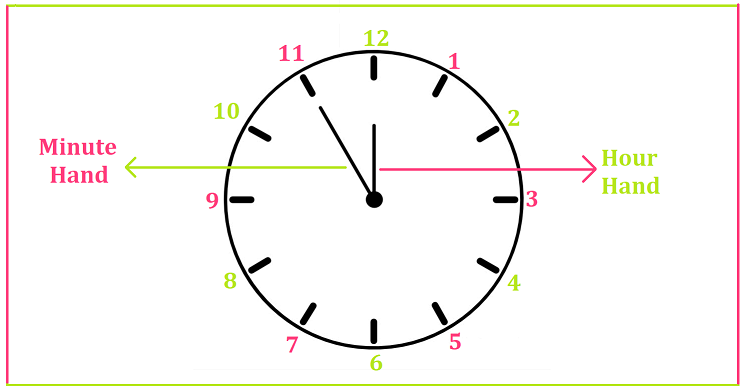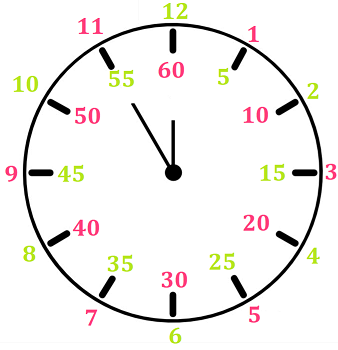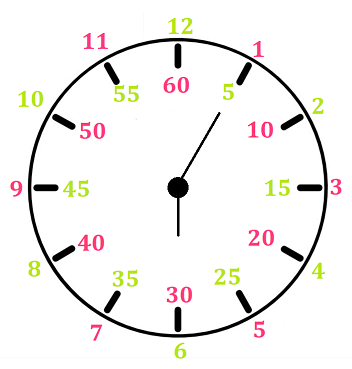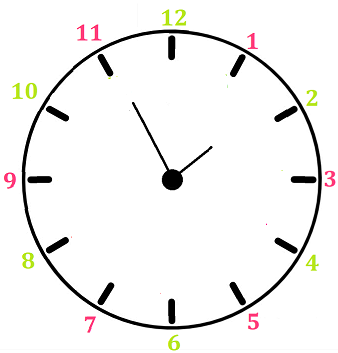# Math > Year 2

## Reading & Drawing time - five minutes interval

A clock has some numbers and some hands.The small hand shows hours.

The large hand shows minutes.

When the minute hand on a clock moves to the next number, it has moved 5 minutes.There are 12 numbers on a clock. The interval of each number is 5 minutes.

So, when the minute hand moves from 1 to the next number, we count up in 5s.

Example 1In the given clock, the hour hand points to 6, and the minute hand points to 1 which means 5 minutes.

The time shown by the clock is 6 hours 5 minutes.

Example 2Here, the hour hand is past 1 and the minute hand points to 11.

The time shown by the clock is 1:55.

//